Anúncio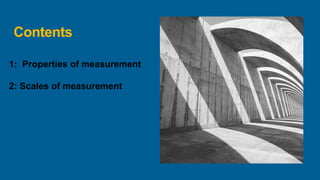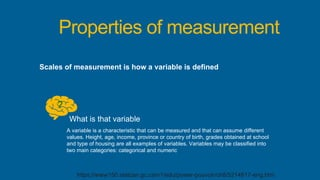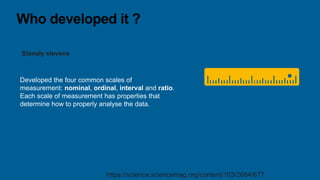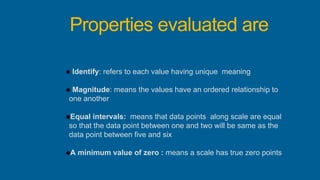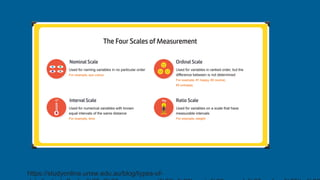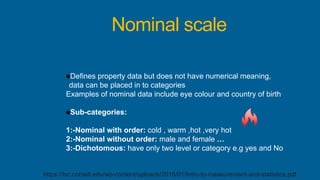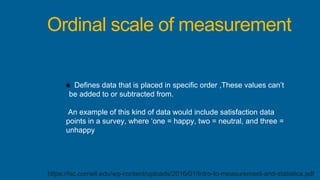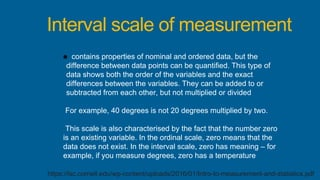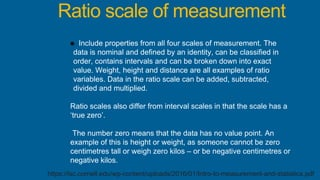1 de 11
Anúncio

### scales of measurement - maneesh jha.pptx

1. Maneesh Jha Scales of measurement Understanding the scales of measurement B.opt 3rd year
2. Contents 1: Properties of measurement 2: Scales of measurement
3. Properties of measurement Scales of measurement is how a variable is defined ? What is that variable A variable is a characteristic that can be measured and that can assume different values. Height, age, income, province or country of birth, grades obtained at school and type of housing are all examples of variables. Variables may be classified into two main categories: categorical and numeric https://www150.statcan.gc.ca/n1/edu/power-pouvoir/ch8/5214817-eng.htm
4. Who developed it ? Stanely stevens https://science.sciencemag.org/content/103/2684/677 Developed the four common scales of measurement: nominal, ordinal, interval and ratio. Each scale of measurement has properties that determine how to properly analyse the data.
5. Properties evaluated are Identify: refers to each value having unique meaning Magnitude: means the values have an ordered relationship to one another Equal intervals: means that data points along scale are equal so that the data point between one and two will be same as the data point between five and six A minimum value of zero : means a scale has true zero points
6. https://studyonline.unsw.edu.au/blog/types-of-
7. Nominal scale Defines property data but does not have numerical meaning, data can be placed in to categories Examples of nominal data include eye colour and country of birth Sub-categories: 1:-Nominal with order: cold , warm ,hot ,very hot 2:-Nominal without order: male and female … 3:-Dichotomous: have only two level or category e.g yes and No https://lsc.cornell.edu/wp-content/uploads/2016/01/Intro-to-measurement-and-statistics.pdf
8. Ordinal scale of measurement Defines data that is placed in specific order ,These values can’t be added to or subtracted from. An example of this kind of data would include satisfaction data points in a survey, where ‘one = happy, two = neutral, and three = unhappy https://lsc.cornell.edu/wp-content/uploads/2016/01/Intro-to-measurement-and-statistics.pdf
9. Interval scale of measurement contains properties of nominal and ordered data, but the difference between data points can be quantified. This type of data shows both the order of the variables and the exact differences between the variables. They can be added to or subtracted from each other, but not multiplied or divided For example, 40 degrees is not 20 degrees multiplied by two. This scale is also characterised by the fact that the number zero is an existing variable. In the ordinal scale, zero means that the data does not exist. In the interval scale, zero has meaning – for example, if you measure degrees, zero has a temperature https://lsc.cornell.edu/wp-content/uploads/2016/01/Intro-to-measurement-and-statistics.pdf
10. Ratio scale of measurement Include properties from all four scales of measurement. The data is nominal and defined by an identity, can be classified in order, contains intervals and can be broken down into exact value. Weight, height and distance are all examples of ratio variables. Data in the ratio scale can be added, subtracted, divided and multiplied. Ratio scales also differ from interval scales in that the scale has a ‘true zero’. The number zero means that the data has no value point. An example of this is height or weight, as someone cannot be zero centimetres tall or weigh zero kilos – or be negative centimetres or negative kilos. https://lsc.cornell.edu/wp-content/uploads/2016/01/Intro-to-measurement-and-statistics.pdf
11. Thank you
Anúncio